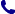• Kontakt
• Newsletter Zapisz się po informacje o nowościach i specjalnych ofertach. Administrator danych: ABE-IPS sp. z o.o.
Wybierz interesujące Cię kategorie
Wyrażam zgodę na przetwarzanie moich danych osobowych w celach marketingowych, tym samym akceptuję Regulamin Newslettera i Politykę prywatności.
Wyrażam zgodę na przesyłanie informacji handlowych drogą elektroniczną.
Wypisz się
• Klienci instytucjonalni
• Dostawa
• O nas
Dostęp on-line

# Książki

0.00 PLN
Schowek (0)
 Schowek jest pusty

## A Statistical Mechanical Interpretation of Algorithmic Information Theory

 Autorzy Wydawnictwo Springer Verlag, Singapore Data wydania 21/11/2019 Liczba stron 136 Forma publikacji książka w miękkiej oprawie Poziom zaawansowania Dla profesjonalistów, specjalistów i badaczy naukowych ISBN 9789811507380 Kategorie Analiza numeryczna, Fizyka statyczna, Fizyka Matematyczna, Algorytmy i struktura danych
244.82 PLN (z VAT)
\$55.07 / £45.57 / €52.49 /
Produkt na zamówienie
Dostawa 3-4 tygodnie
Ilość
Do schowka

## Opis książki

This book is the first one that provides a solid bridge between algorithmic information theory and statistical mechanics. Algorithmic information theory (AIT) is a theory of program size and recently is also known as algorithmic randomness. AIT provides a framework for characterizing the notion of randomness for an individual object and for studying it closely and comprehensively. In this book, a statistical mechanical interpretation of AIT is introduced while explaining the basic notions and results of AIT to the reader who has an acquaintance with an elementary theory of computation. A simplification of the setting of AIT is the noiseless source coding in information theory. First, in the book, a statistical mechanical interpretation of the noiseless source coding scheme is introduced. It can be seen that the notions in statistical mechanics such as entropy, temperature, and thermal equilibrium are translated into the context of noiseless source coding in a natural manner. Then, the framework of AIT is introduced. On this basis, the introduction of a statistical mechanical interpretation of AIT is begun. Namely, the notion of thermodynamic quantities, such as free energy, energy, and entropy, is introduced into AIT. In the interpretation, the temperature is shown to be equal to the partial randomness of the values of all these thermodynamic quantities, where the notion of partial randomness is a stronger representation of the compression rate measured by means of program-size complexity. Additionally, it is demonstrated that this situation holds for the temperature itself as a thermodynamic quantity. That is, for each of all the thermodynamic quantities above, the computability of its value at temperature T gives a sufficient condition for T to be a fixed point on partial randomness. In this groundbreaking book, the current status of the interpretation from both mathematical and physical points of view is reported. For example, a total statistical mechanical interpretation of AIT that actualizes a perfect correspondence to normal statistical mechanics can be developed by identifying a microcanonical ensemble in the framework of AIT. As a result, the statistical mechanical meaning of the thermodynamic quantities of AIT is clarified. In the book, the close relationship of the interpretation to Landauer's principle is pointed out.

A Statistical Mechanical Interpretation of Algorithmic Information Theory

## Spis treści

Statistical Mechanical Interpretation of Noiseless Source Coding.- Algorithmic Information Theory.- Partial Randomness.- Temperature Equals to Partial Randomness.- Fixed Point Theorems on Partial Randomness.- Statistical Mechanical Meaning of the Thermodynamic Quantities of AIT.- The Partial Randomness of Recursively Enumerable Reals.- Computation-Theoretic Clarification of the Phase Transition at Temperature T=1.- Other Related Results and Future Development.

## Polecamy również książki801 777 223